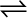7.24 Calculate a) $∆G°$ and b) the equilibrium constant for the formation of NO2 from NO and O2 at 298K

NO (g) + ½ O2 (g)NO2 (g)

where

fG° (NO2) = 52.0 kJ/mol

∆fG° (NO) = 87.0 kJ/mol

∆fG° (O2) = 0 kJ/mol

(a) For the given reaction,
$∆G°$=$∆G°$(Products)-$∆G°$(Reactants)
$∆G°$= 52.0 - {87.0 + 0)
= -35.0 kJ ${\mathrm{mol}}^{-1}$
(b) We know that,
Hence, the equilibrium constant for the given reaction